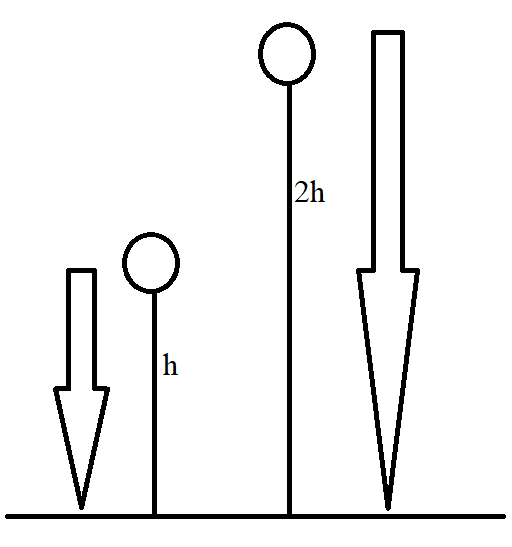QuestionAnswers

# A stone is dropped from a height $h$. It hits the ground with a certain momentum $p$. If the stone is dropped from a height $100\%$ more than the previous height, the momentum when it hits the ground will change byA) $68\%$B) $41\%$C) $200\%$D) $100\%$

Hint: Momentum of a body under free fall refers to the property of the body which determines how much effort is required to stop the body under free fall. Momentum of a body is equal to the product of mass of the body and velocity of the body. Change in momentum refers to the difference between the initial momentum and the final momentum.In the first case, a stone is dropped from a height $h$. From the equations of motion, we can derive that the velocity of the stone under free fall is given by
$v=\sqrt{2gh}$
where
$g$is the acceleration due to gravity and $h$ is the height from which the stone is dropped.
Let this be equation 1.
Also, the momentum of the stone is given by
$p=mv$
where
$p$ is the momentum of the stone
$m$ is the mass of the stone
$v$ is the velocity of the stone
Let this be equation 2.
Substituting equation 2 in equation 1, we have
$p=mv=m\sqrt{2gh}$
Let this be equation 3.
Now, let us move on to the second case. Here, the stone is dropped from a height $100\%$ more than the previous height. If we call this height $H$, it is given by
$H=h+\dfrac{100}{100}h=2h$
Again, if we take the velocity of this stone to be $V$, it is given by
$V=\sqrt{2gH}=\sqrt{2g(2h)}=2\sqrt{gh}$
Let this be equation 4.
Similarly, if the momentum of the stone in the second case is $P$, it is given by
$P=mV=m2\sqrt{gh}$
Let this be equation 5.
Now, to calculate the change in momentum, we subtract equation 3 from equation 5, as follows
$P-p=mV-mv=m2\sqrt{gh}-m\sqrt{gh}=m\sqrt{gh}(\sqrt{2}-1)=0.41m\sqrt{gh}$
Finally, to get the change in momentum in percentage, we take the ratio of this change in momentum to the original momentum and multiply by $100\%$. This is shown as follows.
$\dfrac{P-p}{p}\times 100\%=\dfrac{0.41m\sqrt{gh}}{m\sqrt{gh}}\times 100\%=41\%$

Therefore, the correct option is B.

Note:
Students should note that the same stone is used in both cases. This means that the mass of the stone under free fall remains the same in both cases. Here, it is taken as $m$. If two different stones of different masses are given in the question, students will have to proceed by taking separate values of masses (say ${{m}_{1}}$ and ${{m}_{2}}$).